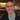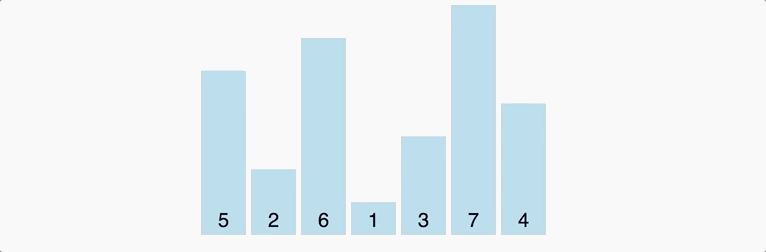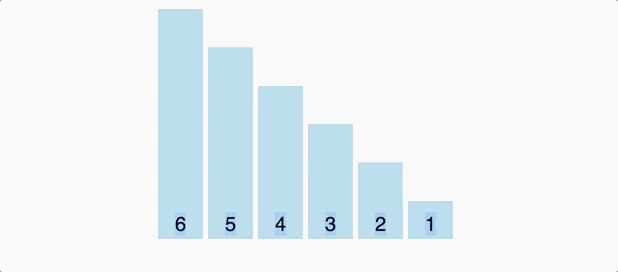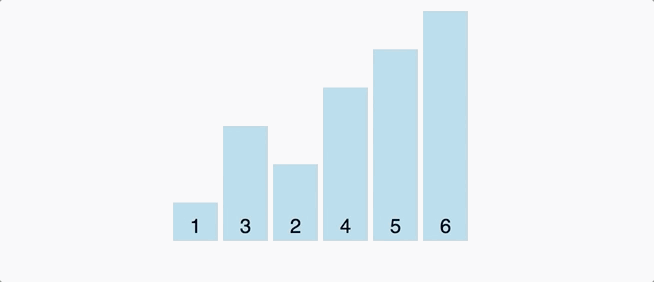# Bubble sort example using Javascript.Written by Thomas Duffy

In this post, we’re going to dive into the bubble sort algorithm using Javascript. The bubble sort algorithm is very straightforward. If given an array of [1,3,5,2,4], the algorithm will walk the collection repeatedly while comparing each item to its next thing and swapping the positions if they’re out of order.

With a time complexity of o(n^2), this sorting algorithm isn’t the most efficient, but it can still be good to know, so when you find yourself in an interview and need a simple brute force solution to sort a small collection, you’ll be set. Let’s get started.

## Inaction

First, let’s look at a visual representation of how this algorithm works in action.animation from visualgo.net

As I mentioned above, we can see bubble sort iterates through the array, comparing and swapping elements repeatedly until sorting is complete. Let’s dig in and write some code to understand better what’s going on here.

### Code example (Working solution)

Bubble sort uses two loops: an outer loop and an inner loop. The inner loop is to compare and swap (bubble up) each item of the array one at a time.

Let’s start with writing just the inner loop.

``````const bubbleSortBF = (array) => {
// inner loop
for (let j = 0; j <= array.length; j++) {
let tempArray;
// check if current item is larger than the to the next item
if (array[j] > array[j + 1]) {
// hold in tempArray cause we're gonna override it when swapping
temp = array[j];
// override current with next position value
array[j] = array[j + 1];
// override next with the temp value we saved earlier.
array[j + 1] = temp;
}
}
return array;
};``````

Now, let’s run the code.animation from visualgo.net

If we execute this code, you’ll see it only walks the array once and doesn’t finish sorting the whole array, and that’s because for the algorithm to finish sorting the entire array, we need to continue iterating through the collection over and over to continue swapping the items until they’re in the correct order. So let’s add the second loop.

``````const bubbleSortBF = (array) => {
// outer loop that restarts the inner loop n times
for (let i = 0; i <= array.length; i++) {
// inner loop that compares and swaps items
for (let j = 0; j <= array.length; j++) {
let temp;
if (array[j] > array[j + 1]) {
// save in temp variable so we can swap items without overriding them
temp = array[j];
// set current index with next position value
array[j] = array[j + 1];
// override next with the temp value we saved earlier.
array[j + 1] = temp;
}
}
}
return array;
};``````

Now lets test our code.animation from visualgo.net

## Optimize code

Earlier, we talked about how this algorithm has a time complexity of o(n^2). This time complexity is because there are nested loops that both can run `n` times to complete the sort.

The code we wrote above is hardcoded to iterate `n` times; This means that our code has a time complexity is o(n^2) no matter if the array is already sorted or not!

I’m sure we can do better. Let’s improve it

## Strategy

To improve this, we can simply add a way to break out or stop the outer loop when the inner loop goes through the array without making any swaps, indicating the array is sorted. Let’s try it.

So one way to break out of the loop early is by using a `do while` loop; This is a control flow statement that allows us to run a block of code over and over again until passed a false boolean.

## Optimized

``````let bubbleSort = (inputArr) => {
let len = inputArr.length;
// swapped will be the flag we use to stop the code execution if the array is sorted.
let swapped;
do {
// we set or reset swapped to false
swapped = false;
// run inner loop
for (let i = 0; i < len; i++) {
// if this check fails for every item in the array, this indicates the array has been sorted,and the swapped flag wont be set to true which will stop code execution
if (inputArr[i] > inputArr[i + 1]) {
let tmp = inputArr[i];
inputArr[i] = inputArr[i + 1];
inputArr[i + 1] = tmp;
// since we made a swap, we can't be sure the total array is sorted yet, so we need to check the array again to make sure all the items are in order. So we set `swapped` to true to trigger the do while to run again
swapped = true;
}
}
} while (swapped);
return inputArr;
};``````

Now let’s test this code by passing in a partially sorted array and make sure it doesn’t operate o(n^2) times.animation from visualgo.net

Awesome! This solution is looking promising. Now, let’s see what happens if we pass this algorithm the worst-case scenario: an array in the reverse order.animation from visualgo.net

As we can see, when passed the worst-case scenario, this algorithm still operates at o(n^2), but that’s ok; this is still much more efficient than our previous implementation.

## conclusion

So that does it for bubble sort. I hope you enjoyed this post and now understand how bubble sort works and how to write it in Javascript. If you liked this post, please feel free to subscribe to my newsletter to get notified when I drop a new post!Written by Thomas Duffy